Nutshell-2019 19th April 2020 HOME "Plato is my friend; Aristotle is my friend, but my greatest friend is truth."- Isaac Newton "Un homme heureux est trop content du présent pour trop se soucier de l'avenir. (A happy man is too satisfied with the present to dwell too much on the future.)" - Albert Einstein "We should consider the laws of Nature as continually changing with the epoch."  - Paul Dirac "Is the purpose of theoretical physics to be no more than a cataloging of all the things that can happen when particles interact with each other and separate? Or is it to be an understanding at a deeper level in which there are things that are not directly observable (as the underlying quantized fields are) but in terms of which we shall have a more fundamental understanding? " - Julian S. Schwinger “In other words, the laws of Newton could be stated not in the form F=ma but in the form: the average kinetic energy less the average potential energy is as little as possible for the path of an object going from one point to another."- Richard P. Feynman "Reading book after book the whole world died, and none ever became learned! But understanding the root matter is what made them gain the knowledge! "- Kabir Granthavali, XXXIII.3, Translated by Charlotte Vaudeville  *** Earlier we met with Aku the Amoeba and his commute in the universe known as “Blip” and as it happened Blip was infested.  There was this quantity which did not change as Bliponians zipped through various Möbius and Lorentz Transformations, a quantity which measured this magnificent infestation, called entropy.       The action-gauge which was supposed to provide information about Blip, was also compromised with entropy.  Which meant Bliponians, were traveling blissfully unaware  on a time-line with only one way forward, straight into a black-hole.   Now Bliponians were not only unaware of entropy but they also did not know that the entropy was continuously increasing with the progression of time.  So if an electron in Blip, was shaken more than 137 times before it spat out a photon.  Then few millions years down the time-line same electron needed to be shaken more than 138 times before a photon popped-out.     It did not matter to Bliponians that their minute existence was progressively sterile.  It did not matter if Bliponians could not count to 2.  But it did matter to some.  Questions were being asked.  The questions which required straight answers, answers that did not come from Bliponian rote, but from the nature herself.  And it was then, the time-line curved away, from its predetermined path to apportion.  It was also then, Blip stopped being a forever-bloating corpse and became a force.         The fundamental  e-γ interaction, represented the measurement-tools the observers were using for their measurements in Blip.  The entropy inherent in the system not only limited the effectiveness of these measurement-tools, but also ensured that these measurement-tools became useless with the progression of time.  The situation was as follows: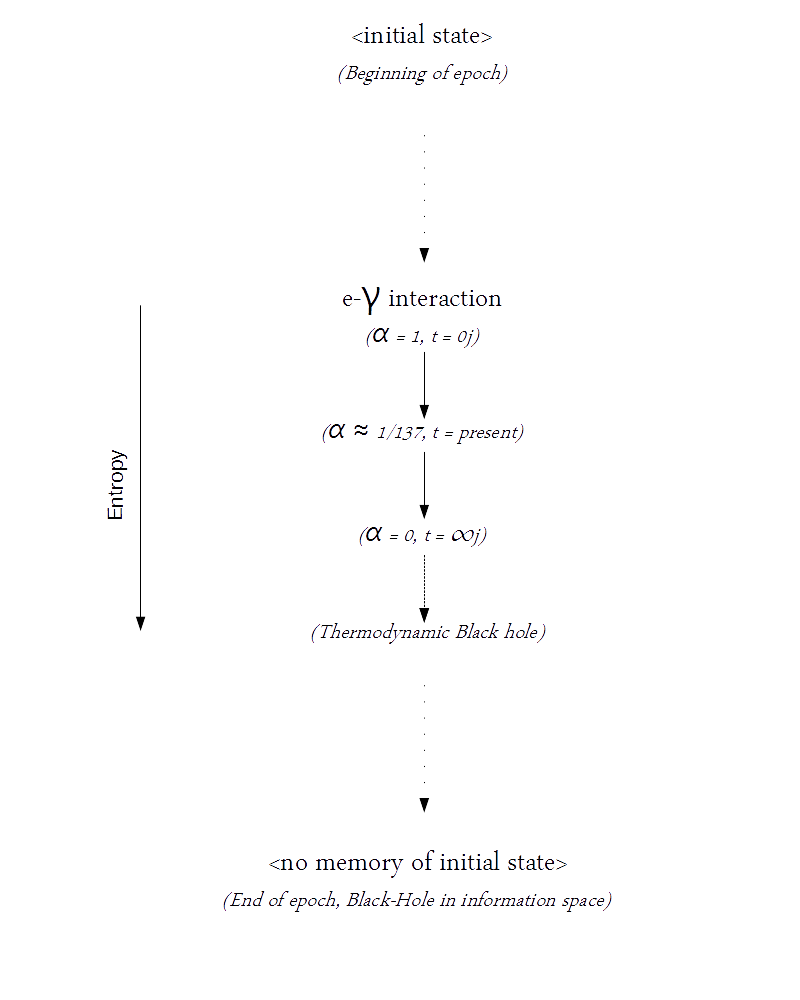The black-hole in j-space, defined the state where the information about the initial state was completely lost, and maximum granularity in measurements was achieved (a.k.a. quantum computer).  This was where the concept of life-force ceased to exist, however an observer in j-space would never be able to measure the exact structure of the black-hole.  In essence, except for the existence of life (or equivalently the initial state), the whole universe was sitting inside a black-hole. Do we need time in Blip?         Whether the time could be slowed down, or a new time-line could be started, or better still if time could be completely eliminated, was not known yet.  But the basic idea was that the underlying topological space by definition, must have no entropy and therefore it must be independent of time.  Therefore the constants defining the fundamental e-γ interaction, must be correlated with each other in a manner such that time did not exist.  Dimensional analysis led to the topological constant: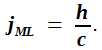Thus any measurement made in any manifold in the Bliponian universe had to follow the topological constraint defined by the relationship above.  The dimensions of the constant jML, were Mass × Length.  No condition was imposed upon the nature of the mass and the length, except for the fact that unlike classical region, they were not independent of each other in the topological space.  Similar constraint was also placed on the fundamental constant h and c, such that in the topological space h and c were not independent of each other.  The relationship shown above, could be written in terms of Compton's Wavelngth as: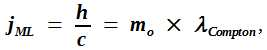where mo was the rest mass.   We could apply the criterion that the time dependence must be eliminated, to get the equivalent topological representation  of a Black-hole (BH) as well.  The Schwarszchild metric in General Theory of Relativity, was given as: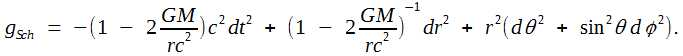Here the coefficient of dt2 term must be zero.  From there the expression for the mass of the BH in topological space, could be derived as: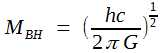, which essentially is the Planck mass.  The estimated mass of a BH was of the order of solar mass, approximately 2 × 1030 kg.  Yet in the topological space, this mass was equivalent to Planck mass, 2.18 × 10-8 kg.  The difference was truly extraordinary.      We can also think of this as duality between the measurements made by ObsM and Obsc.  So the question arose, what did the quantity known as mass really represent?  Was the value of the measured mass an intrinsic property or was it observer dependent?  For a given measurement in j-space, Planck-mass mP, Zero-entropy Mass mZE, and in this case Solar Mass MBH in Kruskal-Szekeres coordinates, were shown below: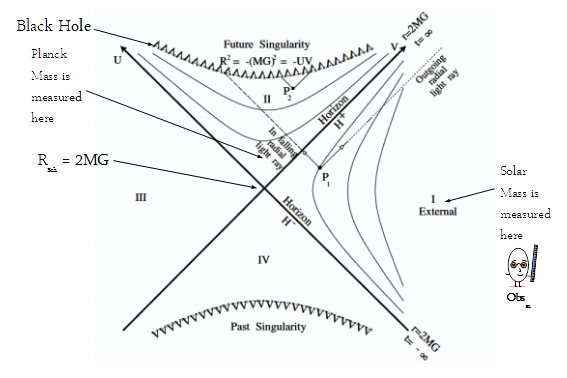Blip existed in the Region-I, so did MBH.  The solar mass MBH was measured by ObsM in the Region-I.  The Planck mass mP was measured in the Region-II and it was measured by Obsc.  The zero-entropy mass mZE corresponded to the mass measured by Obsi.  It was finite but  unmeasurable by either ObsM or Obsc.  (The continuity was defined as the succession of zero-entropy measurements.)      If the radius of Blip was estimated as 4.4×1026 meters, then the definition of the constant jML could be used to estimate the mass of the Blob underlying Blip as 10-67 kg, an incomprehensibly small number.  For comparison the mass of the electron, a Blip entity with no known internal structure, was 9.1×10-31 kg. An ecosystem of  δj-functions:     Consider the internal structure of Blip, without planets, stars, galaxies, BHs etc.  In j-space there was no perfect vacuum as a perfect "null" could not be measured.   For any arbitrary time instant, the fabric in j-space consisted of j-pixels which were seamed together by the Lorentz Invariance i.e the neighbouring j-pixels were bound by Lorentz Invariance.  And since Lorentz Transformation and Möbius Transformation were equivalent to each other, at any given instant on Blip time-line, the structures measured in the neighbouring pixels could be transformed into each other using Möbius transformations.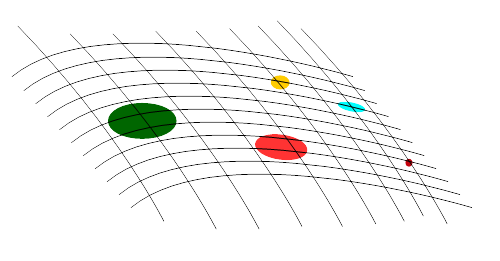The j-space fabric was stretched out in the exterior Region-I.  The remaining regions, II, III, and IV, were beyond ObsM's measurement capabilities.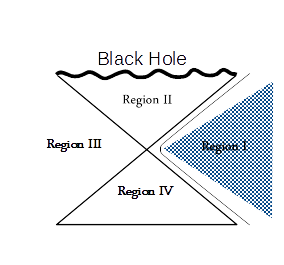So how were the structures introduced into this fabric?  We simply dropped an ecosystem of delta-functions, δj's, into the j-space fabric.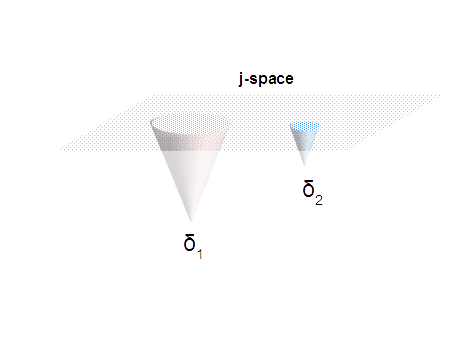It was not as simple though, as the inter-relationships between these delta functions were not understood yet.  However since these delta functions were being measured in j-space and stitched together by Lorentz and Möbius transformations, the definitions of {} (nulls), and the interval [0, 1], were agreed upon by the structures corresponding to δj's.  In other words, the δj's were bound together by the definition of the origin per VT-symmetry in j-space.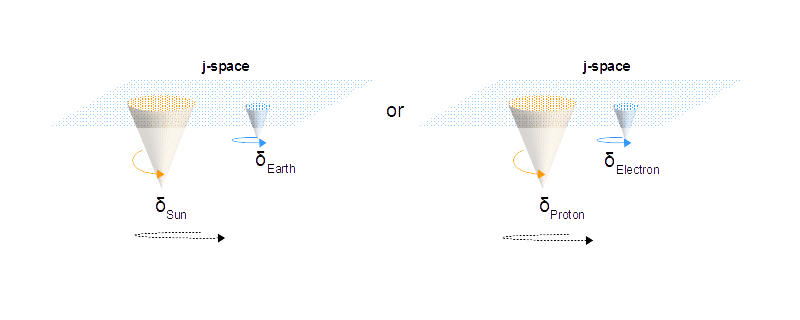Single Element Matrices in j-space:     So how can Aku and his team, think about the field-equation shown below, describing the space-time fabric of Blip, in the topological space?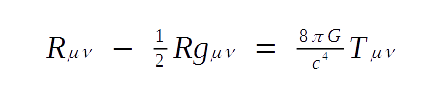The left hand side of the above equation describes the fabric of the j-space in exterior Region-I of KS-coordinates.  Whereas the right hand side of the equation represents resources available to the observer.  Let us consider the metric tensor gμν first.  The metric tensor gμν is a matrix of dimensions m×n, where m and n can take any value up to ∞j,  depending upon the complexity of the system.  However in the topological space, gμν is simply a single element matrix, let us call it Λ.  The single element matrix Λ has some really interesting properties in the discrete measurement space, we call j-space: 1.  Λ is invertible iff it is non-singular. 2.  More importantly Λ is always non-singular in j-space.  The argument is straight forward.  Λ  is singular iff Λ  = .  However in j-space  is equivalent to [0j] which always has a finite value corresponding to the least energy surface and hence Λ is non-singular and invertible both. 3.  Thus the condition that any metric tensor in GR has to be invertible, derives from the requirements of the underlying topological space, and it also prohibits the existence of pure vacuum in j-space since Λ is always finite.  However the condition of invertibility also prohibits the existence of a pure solid as well.  It can be stated as that the condition of "totality" in either direction is prohibited in Blip, per the requirements of the topological space underlying Blip. 4.  Furthermore since Λ is invertible and non-singular, we can derive the condition for  continuity as, B = Λ-1AΛ.  Here A and B are also single-element matrices and hence by definition represent zero-entropy measurements in j-space, and thus continuity i.e. B and A are seamlessly connected via Λ.  (Note that Λ is derived from gμν and it is not a scalar in the topological space.  We are merely counting in the topological space using single-element matrices and Λ.) 5.  In exterior Region-I, Λ can be represented as eiH, where H is a Hermitian matrix.  Since Λ by definition is non-singular, it is not a zero-trace matrix describing a convex surface.  Whereas no such restriction is placed on H, which converges to a zero-trace matrix under the relativistic limits.  Thus eventual metric corresponding to the topological structure is always finite, though it can be represented in terms of  zero-trace matrices in a measurement space corresponding to a lower information content. 6.  The element in single element matrix, is not a number or a scalar per say.  The definition of the element is based on the zero-entropy requirement of the topological space.  The element can be represented as  following:Here the element is shown just as a blob with undetermined characteristics, rather than a number.  The definition of the element is purely a discrete measurement construct.   It represents the limitation of the observer making the measurements.  The element itself can have its own eigenvalue spectrum corresponding to a higher information space, not accessible to either Obsc or ObsM.      Another important issue is the definition of the inverse of the element.  The entities "1" and the operation of inversion, have very different meanings in the topological space, unlike the standard definitions used in exterior region-I of KS representation discussed earlier.  More importantly we must understand, why do we need to perform the inversion operation on the blob, to begin with?  What does this operation signify? Continuity in j-space:         Finally, the concept of continuity exists only in the context of topological space, which actually seems like a "particle" property1.  Traveling on a straight time-line in the Region-I is more analogous to moving in circles but not continuity, and it seems like a "wave" property.  However in the core of all this, is the issue of the "measurement" using a fundamental interaction.  The "measurement" of the topological structure underlying Blip, by using the tools based on a manifold comprising of fundamental interactions such as e-γ and others.  We will discuss single element matrices and the corresponding structures later on.     We have already defined the challenge for ourselves.  We will be discussing the Theory of Special Relativity in the Information Space of an infinite source first.  Then we need to understand the Theory of General Relativity and required Tensor structures.  Penrose diagrams are quite important.   Anharmonic coordinates along with the constructibility condition, represent a powerful tool to describe an interaction.  However all said and done, we must describe the Bliping problem in sufficient details first.  That is all in a Nutshell-2019!     1. An example is the propagation of light through vacuum. ***Previous Blogs: EPR Paradox-I  Nutshell-2015Information on www.ijspace.org is licensed under a Creative Commons Attribution 4.0 International License. Attribution — You must give appropriate credit, provide a link to the license, and indicate if changes were made. You may do so in any reasonable manner, but not in any way that suggests the licensor endorses you or your use. No additional restrictions — You may not apply legal terms or technological measures that legally restrict others from doing anything the license permits. This is a human-readable summary of (and not a substitute for) the license.